# Basic Models of Spin Coating

## S. L. Hellstrom October 28, 2007

### (Submitted as coursework for Physics 210, Stanford University, Autumn 2007)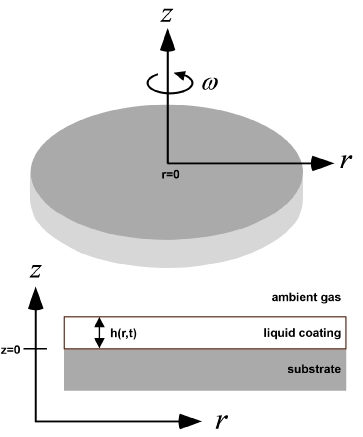Figure 1: Schematic illustrating the spin coating problem, with relevant variables.

## Introduction to Spin Coating

Spin coating is a widespread practice in modern science and engineering, where it is used to deposit uniform coatings of organic materials and/or to uniformly distribute particulate matter on a flat surface. [1,2] In particular, spin coating is used in the microelectronics industry, to coat silicon wafers with a photoresist at the start of the lithographic patterning process.  In order to achieve high-fidelity lithography, a smooth, uniform photoresist film of predictable and reproducible thickness is required.

A great deal of effort has been invested in cataloguing the dependence of film thickness and uniformity on these experimental parameters, especially for coatings of standard photoresists. [4-7] Theoretical analyses of spin coating based on the principles of hydrodynamics have been hindered in part due to the physical and mathematical complexity of the system compared with the relative ease of acquiring process data. Nevertheless even partial analysis can provide some insight into the effectiveness and the limitations of the process.

## Physical Description and Common Approximations

At the most fundamental level the physical system under consideration is that of a viscous fluid on a rotating disk. (Fig. 1) The following approximations are often made: that the rotating plane is horizontal so there is no radial gravitational component; that the liquid layer is so thin that differences in gravitational potential normal to the surface have negligible effect; and that that the radial velocity everywhere is small enough that Coriolis forces can be neglected.

To further simplify the problem, one or more of the following additional approximations may be made: that the rotating plane is infinite; that the liquid layer is radially symmetric; that the liquid is Newtonian; that the shear resistance is only appreciable in horizontal planes; and that the surface is already completely wet. These assumptions are certainly not always justified, however their inclusion provides an effective starting point for a quantitative treatment of the system. While a complete consideration of solvent evaporation in spin coating is complex, it cannot be ignored. It will be introduced to varying degrees of approximation in the following analysis.

## Approximation of No Evaporation

Ignoring evaporation completely, the basic physics were first worked out by Emslie et. al.  By making all of the assumptions described in the previous section, and equating the viscous and centrifugal forces per unit volume, you get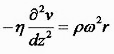where η is the viscosity, ρ the liquid density, ω the spin speed, and v the radial velocity at any point (r, θ, z). For convenience, β=η/ρ defines the kinematic viscosity. Integrating with the boundary conditions that v=0 at the disk surface of z=0, and that dv/dz=0 at the free surface where there cannot be a shearing force, gives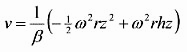From v, radial flow q is easily obtained by integration over z. h is the film thickness. Combining this with continuity equation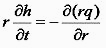gives the differential equation which governs the thickness of the liquid film on the surface as a function of time: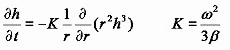As a check, for a uniform initial liquid distribution (h independent of r), the final liquid distribution is also uniform, and a thick layer thins significantly faster than a thin one so there will be a tendency towards uniformity for nonuniform initial films.

Using this last equation and the total derivative expression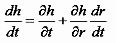one may write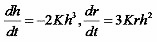with solutions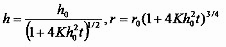These solutions give the coordinates (r, h) of any point on the surface of the disk, in terms of original coordinates (r0, h0). Emslie et. al. analyze the problem further by considering the time and radius dependence of several different initial fluid distributions, and find increasing uniformity with increasing centrifugation time, within the range of validity of the initial set of assumptions. Of course, since there is an infinite disk and no solution evaporation to thicken and solidify the liquid, it will continue to flow radially outwards and thin for ever.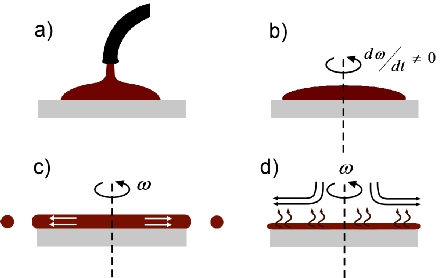Figure 2: The different "stages" of spin coating. a) Dispensation (not modeled). b) Acceleration (not modeled). c) Flow dominated. d) Evaporation dominated.

## Approximation of Constant Evaporation After a Set Point

In order to produce a solid film via spin coating, the solution must evaporate. At the start the concentration of the solute is uniform. The solvent evaporates uniformly over the entire surface area causing a solids concentration c(t) to increase, independently of r since h is independent of r for uniform films. Making the additional approximations i) that c is independent of z, ii) that the volume of the liquid solution is equal to the volume of the solvent plus the volume of the solute, and iii) that the evaporation rate is only a function of experimental parameters such as spin speed, one may define a volume per unit area L of liquid and S of solid, so that c(t)=S/(S+L) and h(t)=S+L. The initial film thickness will reduce uniformly as before, but the thinning rate is different. The rate of change of S and L due to outflow and evaporation at rate e is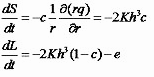These are rates of change of volume per unit area and in theory they can be integrated from initial thickness and concentration values until L=0 and only the solid film remains. However, they cannot be solved analytically.

Meyerhofer solves them numerically, with an empirical relationship between viscosity and concentration of β=βsolventsolids*cγ, with γ around 2.5 for photoresists.  He determines that, if the spin speed is fast enough that the final film thickness does not depend on the initial solution thickness but only on the spin speed - fast enough to generate an outflow-dominated stage that diminishes S0 by 50% or more - then a reasonable approximate solution may be obtained by dividing the spin coating process into two stages. (Fig. 2, c and d) In the first, viscous forces predominantly determine the film thickness. In the second, the film has become thin enough and dense enough that fluid flow essentially stops, and from that point on solvent evaporation dominates. The transition, when the evaporation rate and the viscous flow rate become equal, may be considered the point at which the film "sets" or gels on the substrate. So the initial stage proceeds until the evaporation and outflow contributions are equal: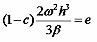with c~c0 and β~β0 since up to this point the solution concentration has been constant. Beyond this point, outflow is negligible, and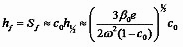Especially for initially thick films and higher spin speeds, this result is a very good approximation to the numerical solution of the full equations with identical parameters.

Viscosity is a particular function of the material being spin coated; however e is not a constant but is proportional to the rate of airflow over the surface, which in turn is (most simply) proportional to the square root of the spin speed.  With this in mind,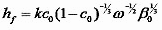with a proportionality constant dependent on the system airflow that must be measured for a given spin coater. The dependence of the final film thickness on the inverse square root of the spin speed is the most common reported experimental relationship between these two quantities,  so for simple systems experiment and theory are already in agreement.

Bornside et. al. in 1991  modify this analysis slightly, to eliminate the empirical constants in the equation. They set the rate of evaporation as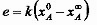where k is the mass transfer coefficient, xA0 is the initial concentration of solvent in the coating liquid, and xA is the mass fraction of solvent in the coating liquid that would be in equilibrium with the mass fraction of solvent in the bulk gas. Then the thickness of the wet film when outflow ceases is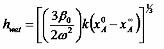and the thickness of the dry film with no liquid that remains at the end of the process is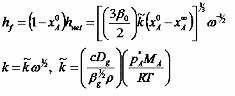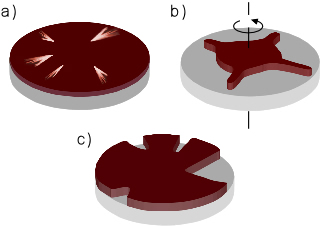Fig. 3: Deviations from the ideal spin coated film. a) Streaks in the film, probably due to particulate matter on the substrate. b) Nonuniform liquid expansion during spinning, due mainly to imperfect substrate wetting. c) Areas of the substrate remain uncoated, which occurs often if the initial liquid volume is too small.

with the dependence on ω pulled out explicitly. c depends on the Schmidt number (the ratio of kinematic viscosity and mass diffusivity) of the ambient gas, Dg is the binary diffusivity of the solvent in the ambient gas, βg is the kinematic viscosity of the ambient gas, pA* is the vapor pressure of the pure solvent at temperature T, and MA is the molecular weight of the solvent.  Bornside et. al. derive this relation from the mass transfer coefficient for transport from the surface of an infinite spinning disk to the bulk of a semi-infinite fluid in laminar flow above it, [11-13] coupled with the relation between vapor pressure and mole fraction in an ideal solution.  They compare this result to experimental data obtained using spin coated poly(methyl methacrylate) dissolved in chloroform, and obtain reasonable agreement with their theoretical results.

## Summary and Conclusion

In sum, the most basic level of analysis is able to demonstrate why spin coating so often reliably produces a film of uniform thickness; it reproduces the usual experimental dependence of film thickness on spin speed; and in simple cases it can predict the final film thickness explicitly based only on process parameters and material properties. Defects and nonuniformities in spin coated films (Fig. 3) ultimately arise from neglected effects that become important in individual cases. One example is particulate dirt on a substrate, which can produce streaking as illustrated in Fig. 3a. For an ab initio investigation of process defects and nonuniformities, one may relax additional assumptions in the theoretical model, typically at the expense of an analytical solution.

For further reading, Acrivos et al. show that uniformity itself is not guaranteed for non-Newtonian liquids.  Moriarty et. al. begin to consider the effects of frontal propagation.  Bornside considers the effects of variation in composition, viscosity and diffusivity as a function of z.  Schwartz et. al. consider the inclusion of viscous, capillary, gravitational, centrifugal, Coriolis and finite contact angle effects, showing for example that one of the main causes of fingers during acceleration (Fig. 3b) is imperfect substrate wetting. 

© 2007 S. L. Hellstrom. The author grants permission to copy, distribute and display this work in unaltered form, with attributation to the author, for noncommercial purposes only. All other rights, including commercial rights, are reserved to the author.

## References

 J. H. Burroughs et al., "Light-Emitting Diodes Based on Conjugated Polymers," Nature, 347, 539 (1990).

 A. Mihi, M. Oca&mtlide;a and H. Míguez, "Oriented Colloidal-Crystal Thin Films by Spin-Coating Microspheres Dispersed in Volatile Media," Adv. Mat., 18, 2244 (2006).

 M. Madou, Fundamentals of Microfabrication: The Science of Miniaturization, 2nd ed.,( CRC Press, 2002).

 J. H. Lai, "An Investigation of Spin Coating of Electron Resists," Polym. Eng. Sci., 19, 1117 (1979).

 B. T. Chen, "Investigation of the Solvent-Evaporation Effect on Spin Coating of Thin Films," Polym. Eng. Sci., 23, 399 (1983).

 W. J. Daughton and F. L. Givens, "An Investigation of the Thickness Variation of Spun-On Thin Films Commonly Associated with the Semiconductor Industry," J. Electrochem. Soc., 129, 173 (1982).

 D. Meyerhofer, "Characteristics of Resist Films Produced by Spinning," J. Appl. Phys., 49, 3993 (1978).

 A. G. Emslie, F. T. Bonner and L. G. Peck, "Flow of a Viscous Liquid on a Rotating Disk", J. Appl. Phys., 29, 5 (1958).

 W. G. Cochran, "The Flow Due to a Rotating Disc", Proc. Cam. Phil. Soc., 30, 365 (1934).

 D. E. Bornside, C. W. Macosko and L. E. Scriven, "Spin Coating of a PMMA/Chlorobenzene Solution", J. Electrochem. Soc., 138, 317 (1991).

 F. Kreith, J. H. Taylor and J. P. Chong, "Heat and Mass Transfer from a Rotating Disk," J. Heat Trans., 81, 95 (1959).

 E. M. Sparrow and J. L. Gregg, "Heat Transfer From a Rotating Disk to Fluids of Any Prandtl Number," J. Heat Trans., 81, 249 (1959).

 ibid., "Mass Transfer, Flow, and Heat Transfer about a Rotating Disk," J. Heat Trans., 82, 294 (1960).

 K. Denbigh, The Principles of Chemical Equilibrium, 4th ed., (Cambridge, 1981).

 A. Acrivos, M. J. Shah and E. E. Petersen, "On the Flow of a Non-Newtonian Liquid on a Rotating Disk," J. Appl. Phys., 31, 963 (1960).

 D. E. Bornside, C. W. Macosko and L. E. Scriven, "Unsteady Spreading of Thin Liquid Films with Small Surface Tension," Phys. Fluids A, 3, 733 (1991).

 J. A. Moriarty, L. W. Schwartz and E. O. Tuck, "Spin Coating: One Dimensional Model," J. Appl. Phys., 66, 5185 (1989).

 L. W. Schwartz and R. V. Roy, "Theoretical and Numerical Results for Spin Coating of Viscous Liquids," Phys. Fluids, 16, 569 (2004).